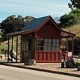# Gas station without pumps

## 2011 October 8

### Physics class progress

The physics class that I’m doing with my son and another home schooler is going a bit slowly.  The other student couldn’t make it again this week (Stanford early admission deadlines, and he needed the time to polish his application essays).

My son and I compared answers to the Chapter 2 problems I’d assigned (see Physics Lab 2).  We’d each made some careless errors (in one, I’d neglected to take a square root, though I’d written the right formula, in another, I’d forgotten to add the sideways movement during acceleration, in a third, my son had forgotten to divide by 2 at one point).  In short, neither of us were doing “A” work—we knew what we were doing, but were being inexcusably sloppy in the computations (me more so than my son). I’ll have to do better on Chapter 3.

I think that I won’t assign any exercises in Chapter 3 this week, to give the other student a chance to catch up.  Instead, I’ll ask my son to finish the data-acquisition code for the ultrasonic rangefinder.  I wrote some simple code running on the Arduino for gathering the data, and he was going to write a Python program (using PySerial) to record the data and output it in a format suitable for plotting with gnuplot.

Our goal is to finish at least the Newtonian mechanics (Chapters 1–13 of Matter and Interactions) before the AP Physics C exam (afternoon of 14 May 2011), which gives us time for a little over 2 weeks per chapter.  That seems like a fairly leisurely pace right now, but perhaps the chapters get harder.  If we finish earlier, it might be worthwhile to do some timed practice with released tests, which are available at AP Central – The AP Physics C: Mechanics Exam. The Mechanics part of the exam is only 90 minutes (it is followed by the other half of Physics C, which we probably will not get to, unless we double our pace). I think that I’ll take the exam along with the two students, risking the embarrassment of them doing better than me.

## Assignment for this week:

• Finish the assignments from the previous posts (the text about how ultrasonic rangefinders work, the problems and program from Chapter 1, the problems and program from Chapter 2).
• For my son only: get the data acquisition program working, so that we can do a lab recording something actually moving.  (We might try video recording it at the same time and comparing Tracker with the ultrasonic rangefinder as a data source.)

## Arduino code for recording from rangefinders:

```// Kevin Karplus
// 30 Sept 2011
//  Using the Ping))) or maxbotix ultrasonic rangefinder
//  to acquire (time_stamp, time_of_flight) pairs and send them over a
//  serial line to a laptop.

// From the documentation:
//   Bidirectional TTL pulse interface on a single I/O pin
//    can communicate with 5 V TTL or 3.3 V CMOS microcontrollers
//   Input trigger: positive TTL pulse, 2 μs min, 5 μs typ.
//   Echo pulse: positive TTL pulse, 115 μs minimum to 18.5 ms maximum.

// As a kluge, the Ping))) is plugged into pins GND,13,12.
// The output pin 13 can provide 40mA of current to the Ping))),
// which only requires about 35mA.

const uint8_t Ping_pin=12;
const uint8_t Vdd_pin=13;

const uint8_t Maxbotix_pin=2;

// speed of sound in air in m/s, as a function of temperature
// in degrees Celsius.
// BUG: currently humidity is ignored, but the speed of sound
//      is higher in more humid air.
inline float speed_sound_t(float temp_C, float relative_humidity=0.0)
{
// return 331.3 + 0.606 *temp_C; // linear approx
return 20.0457 * sqrt(temp_C+273.15);
}

float temp=20.0;  // temperature in degrees Celsius
float speed_sound;  // estimated speed of sound in meters/sec
float half_speed_cm_microsec;  // half the speed of sound in cm/microsecond

void setup()
{   pinMode(Vdd_pin, OUTPUT);
digitalWrite(Vdd_pin, HIGH);
pinMode(Ping_pin, OUTPUT);
digitalWrite(Ping_pin, LOW);

pinMode(Maxbotix_pin, INPUT);
Serial.begin(115200);

speed_sound=speed_sound_t(temp);
half_speed_cm_microsec = speed_sound *0.5 * 100. * 1.e-6;
Serial.print("Assuming temperature of ");
Serial.print(temp);
Serial.print(" gives speed of ");
Serial.print(speed_sound);
Serial.println("m/s");

}

// read_ping returns the time in microseconds for one echo pulse
// There seems to need to be a 1 msec delay needed between read_ping()
// calls (200 microseconds does not seem to be enough).
//
// Reads are very unreliable if the target is close than about 8cm.
digitalWrite(Ping_pin, HIGH);
delayMicroseconds(10);
digitalWrite(Ping_pin, LOW);
// Now there is a 750 microsecond hold off
pinMode(Ping_pin, INPUT);

unsigned long tof= pulseIn(Ping_pin, HIGH);
pinMode(Ping_pin, OUTPUT);
digitalWrite(Ping_pin, LOW);
}

// read_maxbotix returns the time in microseconds for one echo pulse
//
// Reads are very unreliable if the target is close than about 16cm,
// but seem more consistent than the Ping))) for longer distances.b

{
return pulseIn(Maxbotix_pin, HIGH);
}

bool send_Ping_data=0;
bool send_Maxbotix_data=0;
void loop()
{
if (Serial.available())
switch(c)
{  case 'b':
send_Ping_data=1;
send_Maxbotix_data=0;
break;
case 'm':
send_Ping_data=0;
send_Maxbotix_data=1;
break;
case 'e':
send_Ping_data=0;
send_Maxbotix_data=0;
break;
}
}

// For both sensors, report when the measurement was made,
// the time of flight for the pulse to go and return,
// and the computed distance for
// Both are reported in milliseconds.
// The time of measurement is corrected by half the time of flight,
// so that it approximates the time at which the pulse bounced off the object.
if (send_Ping_data)
unsigned long when_measured=micros();
delay(1);   // wait a millisecond to let sensor recover
Serial.print(when_measured-time_of_flight/2);
Serial.print("\t");
Serial.print(time_of_flight);
Serial.print("\t");
Serial.println(time_of_flight*half_speed_cm_microsec);
}
if (send_Maxbotix_data)
unsigned long when_measured=micros();
Serial.print(when_measured-time_of_flight/2);
Serial.print("\t");
Serial.print(time_of_flight);
Serial.print("\t");
Serial.println(time_of_flight*half_speed_cm_microsec);
}

}
```

## 1 Comment »

1.[…] Physics Lab 2 post and Physics class progress described some experiments we were going to do this week with the ultrasonic range […]

Pingback by Physics Lab 3 « Gas station without pumps — 2011 October 14 @ 17:37

This site uses Akismet to reduce spam. Learn how your comment data is processed.